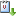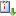Sections
Sie sind hier: Startseite Prof. Eric Renault (CANCELLED!!!)

# Prof. Eric Renault (CANCELLED!!!)

— abgelegt unter:

Identifying Volatility Risk Price through Leverage Effect

Was FDM-Seminar 02.12.2022 von 12:00 bis 13:30 HSII (Alberstr.23b)vCaliCal

In option pricing models with stochastic volatility, changes in volatility affect risk premia through two channels: (1) the investor’s willingness to bear high volatility in order to get high expected returns, as measured by the underlying asset return risk price, and (2) the investor’s direct aversion to changes in future volatility, as measured by the volatility risk price. Disentangling these channels is difficult and poses a subtle identification problem. With a general exponentially affine Stochastic Discount Factor (SDF) model, we focus on theidentification of two risk aversion parameters z1and z2that drive respectively the price of volatility risk and risk aversion to volatility in asset return. Our main theoretical result shows that the presence of leverage effect (instantaneous causality between asset return and its volatility process) reverses two common beliefs regarding the identification of the price z1of volatility risk. First, observations of the variance premium (difference between the risk neutral and historical expectations of the underlying asset return realized variance) may not allow for identification of the price z1of volatility risk. Due to leverage, the variance premium actually depends on both risk aversion parameters z1and z2. Second, observations of the price of the underlying asset (without any option price data) may actually allow for identification of the price of volatility risk, because this risk is latent in the asset return due to leverage. We quantify this identification challenge by adopting the discrete-time exponentially affine model of Han, Khrapov, and Renault (2020), an extension of the idea of “realizing smiles” as proposed by Corsi, Fusari and La Vecchia (2013) for option pricing with data on realized volatility. In particular, we develop a minimum distance criterion that links the asset return risk price, the volatility risk price, and the leverage effect to well-behaved reduced-form parameters that govern the return and volatility’s joint distribution. The link functions are almost flat if the leverage effect is close to zero, raising an issue of (nearly) weak identification of the volatility risk price. We extend the conditional quasi-likelihood ratio test that Andrews and Mikusheva (2016) develop in a nonlinear GMM setting to a minimum distance framework. The resulting conditional quasi-likelihood ratio test is uniformly valid. We invert this test to derive robust confidence sets that provide correct coverage for the risk prices regardless of the leverage effect’s magnitude.

« Juni 2023 »
Juni
MoDiMiDoFrSaSo
1234
567891011
12131415161718
19202122232425
2627282930